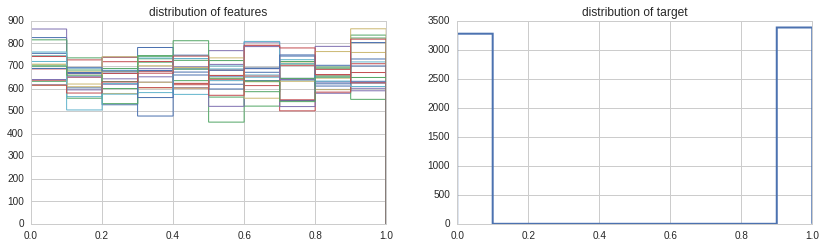Notebook
In :
import pandas as pd
import numpy as np
import sklearn
from matplotlib import pyplot as plt
from sklearn import cross_validation, metrics
from sklearn import linear_model,svm,cluster

In :
org_df = local_csv(u'numerai_training_data_reduced.csv')

In :
for n,name in enumerate(org_df.columns):
if name != 'target':
#plt.figure(n)
#plt.hist(org_df[name],histtype='step',linewidth=1,bins=100)
plt.subplot(221)
plt.hist(org_df[name],histtype='step',linewidth=1,bins=10)
plt.title('distribution of features')
else:
plt.subplot(222)
plt.hist(org_df[name],histtype='step',linewidth=2)
plt.title('distribution of target')### visualize the data¶

#### Y is binary.¶

In :
#numerai.
X = np.array(org_df.drop('target', 1))
Y = np.array(org_df['target'])

In :
X.shape,Y.shape

Out:
((6665, 21), (6665,))
In :
import statsmodels.discrete.discrete_model as sm
logit = sm.Logit(Y, X)
results = logit.fit()
results.summary()

Optimization terminated successfully.
Current function value: 0.689938
Iterations 4

Out:
<caption>Logit Regression Results</caption>
Dep. Variable: No. Observations: y 6665 Logit 6644 MLE 20 Mon, 05 Sep 2016 0.004445 01:50:03 -4598.4 True -4619 0.003658
coef std err z P>|z| [95.0% Conf. Int.] -0.4253 0.205 -2.078 0.038 -0.826 -0.024 -0.4580 0.187 -2.455 0.014 -0.824 -0.092 -0.0439 0.141 -0.311 0.756 -0.321 0.233 0.2892 0.161 1.801 0.072 -0.026 0.604 -0.2264 0.153 -1.477 0.140 -0.527 0.074 0.0234 0.146 0.160 0.873 -0.263 0.310 0.4380 0.215 2.033 0.042 0.016 0.860 -0.1498 0.187 -0.800 0.424 -0.517 0.217 -0.0060 0.128 -0.047 0.962 -0.257 0.245 0.4116 0.177 2.322 0.020 0.064 0.759 0.0765 0.148 0.515 0.606 -0.214 0.367 0.3326 0.154 2.165 0.030 0.031 0.634 0.4307 0.218 1.972 0.049 0.003 0.859 0.1792 0.176 1.019 0.308 -0.165 0.524 -0.5619 0.205 -2.747 0.006 -0.963 -0.161 -0.2112 0.181 -1.164 0.244 -0.567 0.144 -0.4726 0.217 -2.175 0.030 -0.898 -0.047 0.0176 0.160 0.110 0.912 -0.296 0.331 0.3733 0.177 2.112 0.035 0.027 0.720 0.2499 0.148 1.683 0.092 -0.041 0.541 -0.1415 0.189 -0.747 0.455 -0.512 0.230
In :
sk_zoo_list = [('logit regression',linear_model.LogisticRegression),
('kmeans',cluster.KMeans),
('svm',svm.SVC),]

def fit_check(r,model_inst,X_train, X_test, y_train, y_test):
y_pred = model_inst.predict(X_train)
y_true = y_train
acc_train = metrics.accuracy_score(y_true, y_pred)
y_pred = model_inst.predict(X_test)
y_true = y_test
acc_test = metrics.accuracy_score(y_true, y_pred)
print('trial',r,'train acc',acc_train,'test_acc',acc_test)

def iter_fit():
for name,model in sk_zoo_list:
test_size=0.4
print('training',name)
for r in range(3):
random_state=r
X_train, X_test, y_train, y_test = cross_validation.train_test_split(X,Y,test_size=test_size,random_state=random_state)
model_inst = model()
model_inst.fit(X_train,y_train)
fit_check(r,model_inst,X_train, X_test, y_train, y_test)

In :
iter_fit()

('training', 'logit regression')
('trial', 0, 'train acc', 0.53113278319579893, 'test_acc', 0.51575393848462114)
('trial', 1, 'train acc', 0.53463365841460364, 'test_acc', 0.51462865716429107)
('trial', 2, 'train acc', 0.53913478369592394, 'test_acc', 0.51800450112528129)
('training', 'kmeans')
('trial', 0, 'train acc', 0.14828707176794198, 'test_acc', 0.14403600900225055)
('trial', 1, 'train acc', 0.14678669667416855, 'test_acc', 0.13953488372093023)
('trial', 2, 'train acc', 0.12828207051762941, 'test_acc', 0.122655663915979)
('training', 'svm')
('trial', 0, 'train acc', 0.54538634658664664, 'test_acc', 0.51575393848462114)
('trial', 1, 'train acc', 0.54263565891472865, 'test_acc', 0.51762940735183793)
('trial', 2, 'train acc', 0.54263565891472865, 'test_acc', 0.53038259564891221)


results from basic models with default params trained with the reduced dataset seem to indicate X can be used to predict Y.

In :
test_size=0.4
random_state=0
X_train, X_test, y_train, y_test = cross_validation.train_test_split(X,Y,test_size=test_size,random_state=random_state)

In :
from sklearn.linear_model import LogisticRegression
from sklearn.neural_network import BernoulliRBM
from sklearn.grid_search import GridSearchCV
from sklearn.pipeline import Pipeline
import time

In :
dir(sklearn.neural_network)

Out:
['BernoulliRBM', 'rbm']
In :
# ref
# http://scikit-learn.org/stable/auto_examples/neural_networks/plot_rbm_logistic_classification.html#example-neural-networks-plot-rbm-logistic-classification-py
# http://www.pyimagesearch.com/2014/06/23/applying-deep-learning-rbm-mnist-using-python/

verbose = 0
rbm = BernoulliRBM(verbose=verbose)
rbm2 = BernoulliRBM(verbose=verbose)
logistic = LogisticRegression()

classifier = Pipeline([("rbm", rbm), ("logistic", logistic)])
#classifier = Pipeline([("rbm", rbm), ("rbm2", rbm2),("logistic", logistic)])

print "SEARCHING RBM + LOGISTIC REGRESSION"
params = {
"rbm__learning_rate": [0.01,],
"rbm__n_components": [25,50,100],
"rbm__batch_size": [10,20],
"rbm__n_iter": ,
"logistic__C": [0.1],
#"rbm2__learning_rate": [0.01],
#"rbm2__n_components": [4,8],
#"rbm2__n_iter": ,
}
start = time.time()
# perform a grid search over the parameter
gs = GridSearchCV(classifier, params, n_jobs=10, verbose = 1)
gs.fit(X_train,y_train)

# print diagnostic information to the user and grab the
# best model
print "\ndone in %0.3fs" % (time.time() - start)
print "best score: %0.3f" % (gs.best_score_)
print "RBM + LOGISTIC REGRESSION PARAMETERS"
bestParams = gs.best_estimator_.get_params()

# loop over the parameters and print each of them out
# so they can be manually set
for p in sorted(params.keys()):
print "\t %s: %f" % (p, bestParams[p])

SEARCHING RBM + LOGISTIC REGRESSION
Fitting 3 folds for each of 6 candidates, totalling 18 fits

done in 866.001s
best score: 0.517
RBM + LOGISTIC REGRESSION PARAMETERS
logistic__C: 0.100000
rbm__batch_size: 20.000000
rbm__learning_rate: 0.010000
rbm__n_components: 25.000000
rbm__n_iter: 1000.000000

In :
fit_check(None,gs,X_train, X_test, y_train, y_test)

('trial', None, 'train acc', 0.52463115778944736, 'test_acc', 0.51687921980495122)

In [ ]:
#print(dir(gs.best_estimator_.named_steps['rbm']))


#### dataset¶

Like most folks are saying in the thread (https://www.quantopian.com/posts/implementing-and-launching-deep-learning-algo )... it is a bit anonoying not knowing what the features and target are. Without knowing what they are, the only thing you are left to, blindly, play with are model selection and parameter tuning. Data preprocessing is likely unnecessary, since it is likely already processed, given that it is uniformly distributed, or even dangerous, since you have no a priori knowledge on what those features are.

#### sklearn neural net package¶

sklearn does not have dropout, relu activation, which have been shown to improve training for deep nets (more nodes,more layers). also, i can't seem to locate the loss per iteration. so you don't really know if with each iteration, the model is learning or not.

In [ ]: